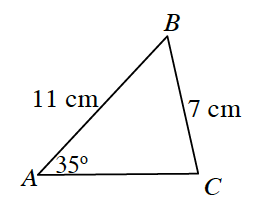### Home > PC3 > Chapter 10 > Lesson 10.2.2 > Problem10-129

10-129.

Solve for all possible triangles given $AB = 11$ cm, $BC = 7$ cm, and $A = 35º$.

Draw a diagram.Since the given information is SSA, use the Law of Cosines to help you determine the number of possible triangles.